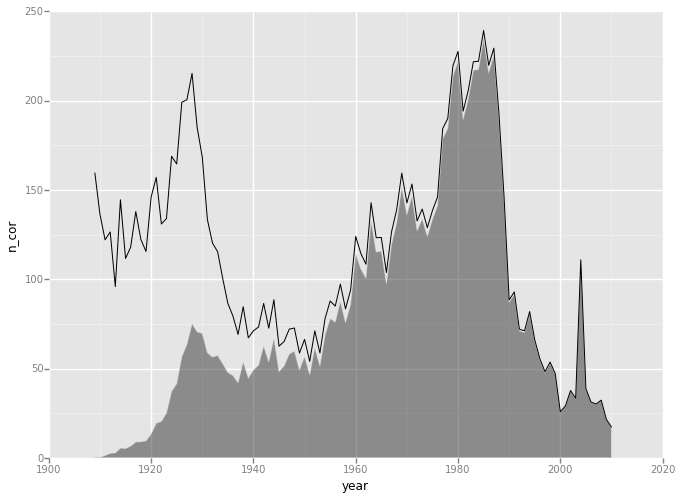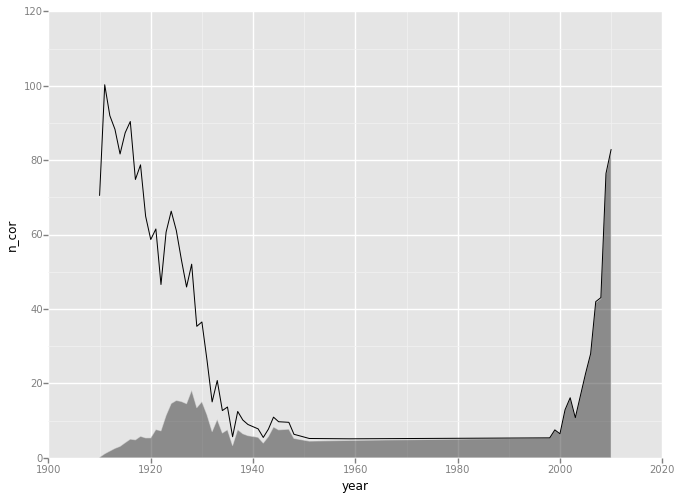# Relating Age to Name¶

This notebook is inspired by Nate Silver's recent article on How to Tell Someone's Age When All you Know is Her Name. It allows one to (almost) replicate the analysis done in the article, and provides more extensive features. I have done similar work using R, and you can find it here.

## Data¶

We will uses four primary datasets.

In :
%matplotlib inline
import re
import urllib
from zipfile import ZipFile
from path import path
import numpy as np
import pandas as pd
from scipy.interpolate import interp1d
import matplotlib.pyplot as plt
import seaborn as sns
from ggplot import *

/Users/ramnathv/anaconda/lib/python2.7/site-packages/pytz/__init__.py:29: UserWarning: Module argparse was already imported from /Users/ramnathv/anaconda/lib/python2.7/argparse.pyc, but /Users/ramnathv/anaconda/lib/python2.7/site-packages is being added to sys.path
from pkg_resources import resource_stream


### Baby Names¶

In :
urllib.urlretrieve("http://www.ssa.gov/oact/babynames/names.zip", "names.zip")
zf = ZipFile("names.zip")
data['year'] = int(re.findall(r'\d+', f))
return data

bnames = pd.concat([read_names(f) for f in zf.namelist() if f.endswith('.txt')])

Out:
name sex n year
0 Mary F 9217 1884
1 Anna F 3860 1884
2 Emma F 2587 1884
3 Elizabeth F 2549 1884
4 Minnie F 2243 1884

5 rows × 4 columns

### Baby Names by State¶

In :
urllib.urlretrieve("http://www.ssa.gov/oact/babynames/state/namesbystate.zip", "namesbystate.zip")
zf = ZipFile("namesbystate.zip")
return pd.read_csv(zf.open(f), header = None, names = ['state', 'sex', 'year', 'name', 'n'])

bnames_by_state = pd.concat([read_names2(f) for f in zf.namelist() if f.endswith('.TXT')])

Out:
state sex year name n
0 MA F 1910 Mary 988
1 MA F 1910 Helen 473
2 MA F 1910 Margaret 374
3 MA F 1910 Dorothy 331
4 MA F 1910 Alice 313

5 rows × 5 columns

### Cohort Life Tables¶

The next dataset is actuarial cohort life tables provided by SSA. I was unable to figure out how to scrape this data using BeautifulSoup. So I used the R package XML to scrape these lifetables and saved it to lifetables.csv. If you are interested in the R code, you can find it here.

In :
lifetables = pd.read_csv('lifetables.csv')

Out:
x qx lx dx Lx Tx ex sex year
0 0 0.14596 100000 14596 90026 5151511 51.52 M 1900
1 1 0.03282 85404 2803 84003 5061484 59.26 M 1900
2 2 0.01634 82601 1350 81926 4977482 60.26 M 1900
3 3 0.01052 81251 855 80824 4895556 60.25 M 1900
4 4 0.00875 80397 703 80045 4814732 59.89 M 1900

5 rows × 9 columns

You can read the documentation for the lifetables to understand the various parameters. The key column of interest to us is lx, which provides the number of people born in the year year who live upto the age x. Since we are in the year 2014, we are only interested in a subset of the data.

In :
lifetables_2014 = lifetables[lifetables['year'] + lifetables['x'] == 2014]

Out:
x qx lx dx Lx Tx ex sex year
114 114 0.76365 0 0 0 0 0.79 M 1900
234 114 0.75783 0 0 0 0 0.80 F 1900
344 104 0.46882 25 12 19 38 1.54 M 1910
464 104 0.42317 191 81 150 328 1.72 F 1910
574 94 0.26858 3122 838 2703 8656 2.77 M 1920

5 rows × 9 columns

The cohort life tables are provided only for every decade. Since we need the data by year, we will use spline interpolation to fill out the gaps.

In :
def process(d, kind = 'slinear'):
f = interp1d(d.year, d.lx, kind)
year = np.arange(1900, 2011)
lx = f(year)
return pd.DataFrame({"year": year, "lx": lx, "sex": d.sex.iloc})
lifetable_2014 = lifetables_2014.\
groupby('sex', as_index = False).\
apply(process)

Out:
lx sex year
0 0.0 F 1900
1 19.1 F 1901
2 38.2 F 1902
3 57.3 F 1903
4 76.4 F 1904

5 rows × 3 columns

### Census Live Births Data¶

Finally, we need live births data from the census to extrapolate the birth data to account for the correct that not all births were recorded by SSA till around 1930, since it wasn't mandatory.

In :
urllib.urlretrieve("http://www.census.gov/statab/hist/02HS0013.xls", "02HS0013.xls")
dat = pd.read_excel('02HS0013.xls', sheetname = 'HS-13', skiprows = range(14))
tot_births = dat.ix[9:101,:2].reset_index(drop = True)
tot_births.columns = ['year', 'births']
tot_births = tot_births.convert_objects(convert_numeric = True)

Out:
year births
0 1909 2718
1 1910 2777
2 1911 2809
3 1912 2840
4 1913 2869

5 rows × 2 columns

We will now merge this data with the bnames aggregated by year to compute a set of correction factors, which will be used to scale the number of births. I have taken a very naive approach to do this, and there might be better ways to accomplish the same.

In :
cor_factors = bnames.\
groupby('year', as_index = False).\
sum().\
merge(tot_births)
cor_factors['cor'] = cor_factors['births']*1000/cor_factors['n']
cor_factors = cor_factors[['year', 'cor']]

Out:
year cor
0 1909 5.316662
1 1910 4.701138
2 1911 4.360075
3 1912 2.874415
4 1913 2.523176

5 rows × 2 columns

We expand the correction factors data for the years 2002 to 2014 using the correction factor for the year 2001.

In :
cor_new = pd.DataFrame({
'year': range(2002, 2014),
'cor': cor_factors.cor.iloc[-1]
})
cor_factors = pd.concat([cor_factors, cor_new])[['year', 'cor']]

Out:
year cor
0 1909 5.316662
1 1910 4.701138
2 1911 4.360075
3 1912 2.874415
4 1913 2.523176

5 rows × 2 columns

## Analysis¶

Now that we have all the required data, we need a few helper functions to help us with our analysis. The first function we will write is get_data, which takes name, sex and state and returns a data frame with the distribution of number of births and number of people alive by year.

In :
def get_data(name, sex, state = None):
if state is None:
dat = bnames
else:
dat = bnames_by_state[(bnames_by_state["state"] == state)]
data = dat[(dat['name'] == name) & (dat['sex'] == sex)].\
merge(cor_factors).\
merge(lifetable_2014)
data['n_cor'] = data['n']*data['cor']
data['n_alive'] = data['lx']/(10**5)*data['n_cor']
return data

Out:
name sex n year cor lx n_cor n_alive
0 Violet F 945 1909 5.316662 171.9 5024.245779 8.636678
1 Violet F 1037 1910 4.701138 191.0 4875.080412 9.311404
2 Violet F 1183 1911 4.360075 1084.2 5157.968506 55.922695
3 Violet F 1535 1912 2.874415 1977.4 4412.227601 87.247389
4 Violet F 1751 1913 2.523176 2870.6 4418.081208 126.825439

5 rows × 8 columns

Our next helper function is plot_name which accepts the same arguments as get_data, but returns a plot of the distribution of number of births and number alive by year.

In :
def plot_name(name, sex, state = None):
data = get_data(name, sex, state)
return ggplot(data, aes('year', 'n_cor')) +\
geom_line() +\
geom_area(aes(ymin = 0, ymax = 'n_alive'), alpha = 0.5)
plot_name("Joseph", "F")Out:
<ggplot: (279099421)>
In :
plot_name("Violet", "F", "MA")Out:
<ggplot: (279096937)>

### Estimate Age¶

We will now write a function that will help us figure out the probability that a person with a certain name is alive, as well as the quantiles of their age distribution. Since we are dealing with weighted data, I will use some code copied from the wquantiles module. The quantile function accepts a data array and a weights array to return a specific quantile

In :
# from the module wquantiles https://github.com/nudomarinero/wquantiles/blob/master/weighted.py
import numpy as np
def quantile_1D(data, weights, quantile):
"""
Compute the weighted quantile of a 1D numpy array.

Parameters
----------
data : ndarray
Input array (one dimension).
weights : ndarray
Array with the weights of the same size of data.
quantile : float
Quantile to compute. It must have a value between 0 and 1.

Returns
-------
quantile_1D : float
The output value.
"""
# Check the data
if not isinstance(data, np.matrix) :
data = np.asarray(data)
if not isinstance(weights, np.matrix) :
weights = np.asarray(weights)
nd = data.ndim
if nd != 1:
raise TypeError("data must be a one dimensional array")
ndw = weights.ndim
if ndw != 1:
raise TypeError("weights must be a one dimensional array")
if data.shape != weights.shape:
raise TypeError("the length of data and weights must be the same")
if ((quantile > 1.) or (quantile < 0.)):
raise ValueError("quantile must have a value between 0. and 1.")
# Sort the data
ind_sorted = np.argsort(data)
sorted_data = data[ind_sorted]
sorted_weights = weights[ind_sorted]
# Compute the auxiliary arrays
Sn = np.cumsum(sorted_weights)
# TODO: Check that the weights do not sum zero
Pn = (Sn-0.5*sorted_weights)/np.sum(sorted_weights)
# Get the value of the weighted median
return np.interp(quantile, Pn, sorted_data)

def quantile(data, weights, quantile):
"""
Weighted quantile of an array with respect to the last axis.

Parameters
----------
data : ndarray
Input array.
weights : ndarray
Array with the weights. It must have the same size of the last
axis of data.
quantile : float
Quantile to compute. It must have a value between 0 and 1.

Returns
-------
quantile : float
The output value.
"""
# TODO: Allow to specify the axis
nd = data.ndim
if nd == 0:
TypeError("data must have at least one dimension")
elif nd == 1:
return quantile_1D(data, weights, quantile)
elif nd > 1:
n = data.shape
imr = data.reshape((np.prod(n[:-1]), n[-1]))
result = np.apply_along_axis(quantile_1D, -1, imr, weights, quantile)
return result.reshape(n[:-1])


We will use the quantile function to write an estimate_age function that will return the living probabilities and quantiles for a given name, sex and state.

In :
def estimate_age(name, sex, state = None):
data = get_data(name, sex, state)
qs = [1, 0.75, 0.5, 0.25, 0]
quantiles = [2014 - int(quantile(data.year, data.n_alive, q)) for q in qs]
result = dict(zip(['q0', 'q25', 'q50', 'q75', 'q100'], quantiles))
result['p_alive'] = round(data.n_alive.sum()/data.n_cor.sum()*100, 2)
result['sex'] = sex
result['name'] = name
return pd.Series(result)

estimate_age('Gertrude', 'F')

Out:
name       Gertrude
p_alive       18.17
q0                4
q100            105
q25              71
q50              82
q75              90
sex               F
dtype: object
In :
estimate_age('Ava', 'F')

Out:
name         Ava
p_alive    95.24
q0             4
q100         105
q25            6
q50            8
q75           10
sex            F
dtype: object

We can now use the estimate_age function to compute the quantiles for the most common names and replicate the plots in the Nate Silver article.

In :
top_100_names = bnames.\
groupby(['name', 'sex'], as_index = False).\
sum().\
sort('n', ascending = False)
top_25_females = top_100_names[(top_100_names["sex"] == "F")]
estimates = pd.concat([estimate_age(name, 'F') for name in top_25_females["name"].iloc[:25].tolist()], axis = 1)
estimates.T.sort('q50').reset_index()

Out:
index name p_alive q0 q100 q25 q50 q75 sex
0 24 Emily 90.62 4 105 12 19 28 F
1 18 Ashley 98.5 4 97 18 24 28 F
2 10 Jessica 97.9 4 105 22 27 32 F
3 9 Sarah 83.63 4 105 19 28 37 F
4 17 Anna 51.81 4 105 17 34 65 F
5 3 Jennifer 96.63 4 98 29 36 42 F
6 1 Elizabeth 70.05 4 105 23 39 58 F
7 23 Michelle 95.92 4 99 29 40 47 F
8 20 Kimberly 95.87 4 81 30 41 48 F
9 15 Lisa 94.48 4 104 40 47 51 F
10 14 Karen 89.12 4 105 48 55 62 F
11 7 Susan 86.52 4 105 50 57 63 F
12 19 Donna 81.85 4 105 52 58 66 F
13 16 Sandra 85.46 4 105 50 59 67 F
14 12 Nancy 76.02 4 105 53 62 70 F
15 2 Patricia 77.8 4 105 53 62 70 F
16 4 Linda 84.87 4 105 55 62 67 F
17 22 Carol 77.49 4 105 56 64 71 F
18 0 Mary 51.35 4 105 53 64 75 F
19 5 Barbara 71.56 4 105 57 65 73 F
20 6 Margaret 45.63 4 105 51 65 76 F
21 21 Ruth 34.23 4 105 57 70 83 F
22 13 Betty 50.7 4 105 65 74 82 F
23 11 Helen 29.38 4 105 61 74 86 F
24 8 Dorothy 34.24 4 105 64 76 85 F

25 rows × 9 columns

We can go a step beyond the article and also use the state parameter to get more specific quantiles, if we know the place of birth of a person.

The final step for me is to convert all of this into an interactive webapp. I have done it with R using the shiny package. I am still getting the hang of web frameworks in python, so it will be a while before I get to doing this.